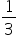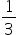Mathematics
Easy

Question

# If DF = 9y + 4, BC= 3y + 13, what is the length of DF?

## 5789Hint:

## The correct answer is: 7

### Step 1 of 1:We have given If DF = 9y + 4, BC= 3y + 13BC = 2DF3y + 13 = 2(9y + 4)3y + 13= 18y + 815y = 5y =DF = 9y + 4 = 9 () + 4= 3 + 4 = 7

Linear equation in one variable can be solved using fundamental operations

### Related Questions to study#### With Turito Foundation.#### Get an Expert Advice From Turito.